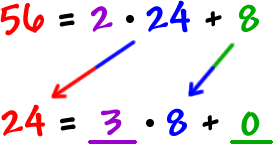# Notes of Number Theory by Umer AsgharThese notes are very helpful to prepare one of the sections paper of mathematics for BSc.

 Author: Umer Asghar Composed PDF (1.14 mB) 24
• Divisibility
• Division algorithm or Euclid theorem
• Application of division algorithm
• Mathematical induction
• Greatest common divisor
• Linear combination
• Relatively prime
• Alternative definition of G.C.D
• Least common multiple
• Alternative definition of L.C.M

• bsc/notes-of-number-theory-by-umer-asghar